# Introducing GAs without Phenotype

A reduced formalization of the above idea of genetic inheritance as genetic algorithm without phenotype is described below. It assumes a populationas a set of individual elements called agents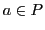and as a minimal property of an agent it is assumed that he possesses genetic informations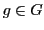where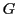is a subset of binary strings of a fixed size4.3.

With these assumptions one can define a simple algorithm to simulate genetic inheritance.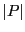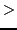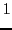(4.1)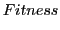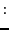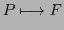(4.2)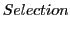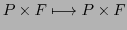(4.3)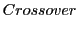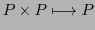(4.4)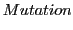(4.5)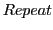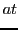(4.6)

Formula 4.1 assumes that there is an initial populationwith at least two members which can produce offspring. If there is a populationthen can the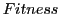-function compute some fitness valuefor each agent of the population. The fitness function as such is no part of the GA; it has to be 'provided' by the user of the GA. If there is a population with fitness values for each agent then it is possible to select a subset out of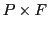. Usually this subset takes these members ofwhich have the highest fitness values. Different to real populations are virtual populations understood as staying constant in number. If there are no more members for such a selection then the populationis extincted. Otherwise the GA algorithm continues with 'playing' with the genetic information. There are two main options: (i) keeping the given structures but arrange them in a new way (one example is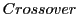mixing the genetic informations of two different agents by keeping the different parts 'as they are'); (ii) changing the given structures in a 'new' way (one example is called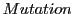by changing some part of the given information). After these Modifications the original populationhas changed to a new format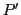, which is the starting point for a new cycle of the GA algorithm.

There arise many deep questions regarding mathematical properties of such a GA algorithm. Before these will be discussed some examples will be presented4.4.

The following example is inspired by Goldberg's famous book from 1989, chapters 1-2. For the software used in this example look to the section entitled Programs. There are different versions. The usage of the different versions is described at the beginning of the section Programs.

Subsections
Gerd Doeben-Henisch 2013-01-14